# Variable selection for the Gambia data

### Chapter 5.3: Stochastic search variable selection

The gambia data in the geoR package includes data for 1332 children in the Gambia. The binary response $$Y_i$$ is the indictor that child $$i$$ tested positive for malaria. We use five covariates in $$X_{ij}$$:

1. Age: age of the child, in days

2. Netuse: indicator variable denoting whether (1) or not (0) the child regularly sleeps under a bed-net

3. Treated: indicator variable denoting whether (1) or not (0) the bed-net is treated (coded 0 if netuse=0)

4. Green: satellite-derived measure of the greenness of vegetation in the immediate vicinity of the village (arbitrary units)

5. PCH: indicator variable denoting the presence (1) or absence (0) of a health center in the village

We use the logit regression model $\mbox{logit}[\mbox{Prob}(Y_i=1)) = \alpha + \sum_{j=1}^pX_{ij}\beta_j.$ The spike-and-slab prior for $$\beta_j$$ is $$\beta_j=\gamma_j\delta_j$$ where $$\gamma_j\sim\mbox{Bernoulli}(0.5)$$ and $$\delta_j\sim\mbox{Normal}(0,\tau^2)$$.

library(geoR)

Y <- gambia[,3]
X <- gambia[,4:8]

Y[1:5]

##  1 0 0 1 0

X[1:5,]

##       age netuse treated green phc
## 1850 1783      0       0 40.85   1
## 1851  404      1       0 40.85   1
## 1852  452      1       0 40.85   1
## 1853  566      1       0 40.85   1
## 1854  598      1       0 40.85   1

names <- c("Age","Netuse","Treated","Green","PCH")
for(j in 1:5){
boxplot(X[,j]~Y,main=names[j])
}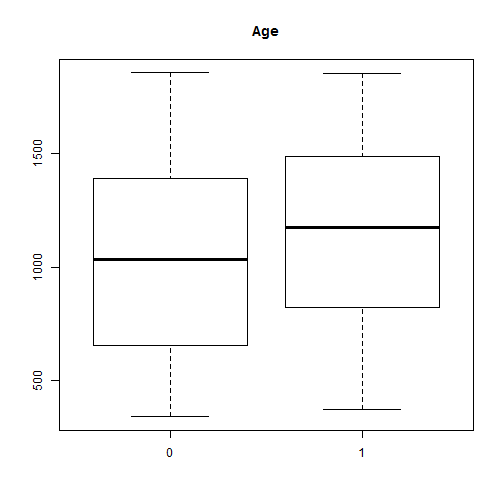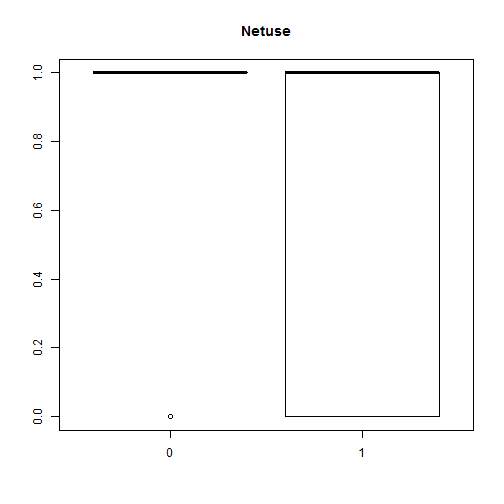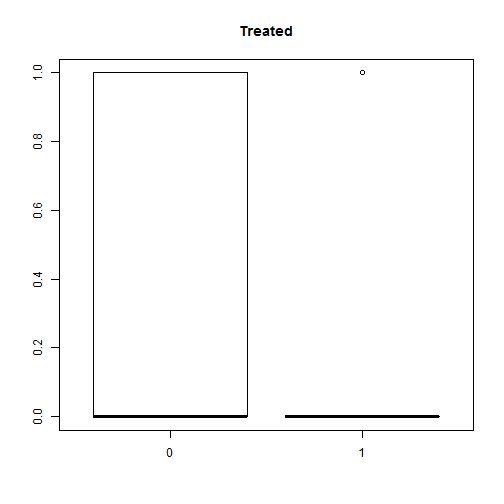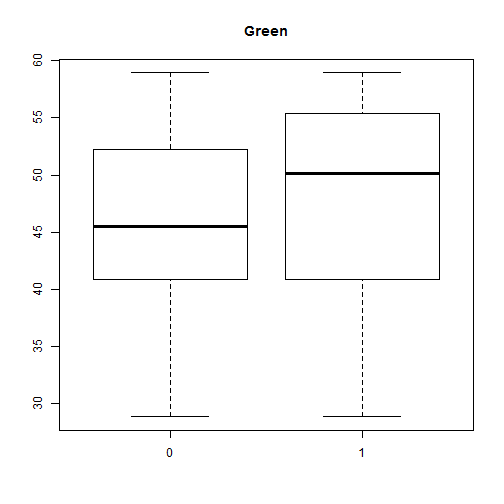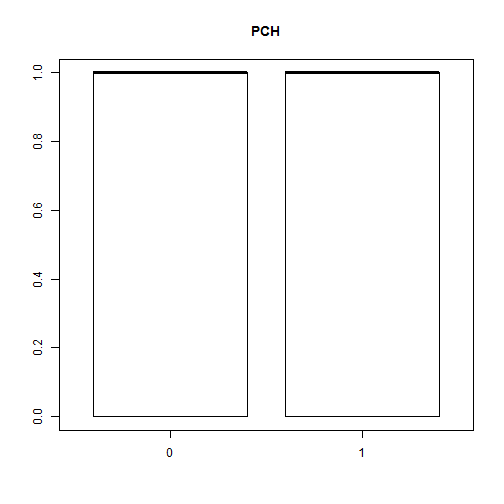# Standardize X
X <- scale(X)
X[1:5,]

##            age     netuse    treated      green       phc
## 1850  1.650148 -1.5683351 -0.6167739 -0.8462609 0.6802564
## 1851 -1.588791  0.6373055 -0.6167739 -0.8462609 0.6802564
## 1852 -1.476050  0.6373055 -0.6167739 -0.8462609 0.6802564
## 1853 -1.208292  0.6373055 -0.6167739 -0.8462609 0.6802564
## 1854 -1.133132  0.6373055 -0.6167739 -0.8462609 0.6802564

n <- length(Y)
p <- ncol(X)


## Define the models in JAGS

   library(rjags)

m <- textConnection("model{
for(i in 1:n){
Y[i]          ~ dbern(pi[i])
logit(pi[i]) <- alpha          + X[i,1]*beta + X[i,2]*beta +
X[i,3]*beta + X[i,4]*beta + X[i,5]*beta
}
for(j in 1:5){
beta[j] <- gamma[j]*delta[j]
gamma[j] ~ dbern(0.5)
delta[j] ~ dnorm(0,tau)
}
alpha ~ dnorm(0,0.01)
tau   ~ dgamma(0.1,0.1)
}")


## Fit the model

   data   <- list(Y=Y,X=X,n=n)
burn   <- 10000
iters  <- 50000
chains <- 3
model  <- jags.model(m,data = data, n.chains=chains,quiet=TRUE)
update(model, burn, progress.bar="none")
samps  <- coda.samples(model, variable.names=c("beta"),
thin=5, n.iter=iters, progress.bar="none")
plot(samps)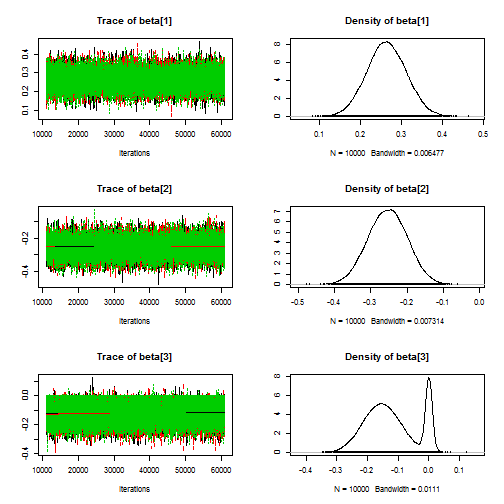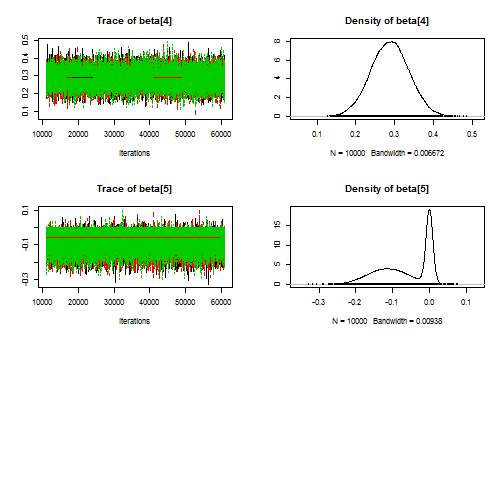## Summarize the marginal distributions of the $$\beta_j$$

   beta    <- NULL
for(l in 1:chains){
beta <- rbind(beta,samps[[l]])
}
colnames(beta) <- names

for(j in 1:5){
hist(beta[,j],xlab=expression(beta[j]),ylab="Posterior density",
breaks=100,main=names[j])
}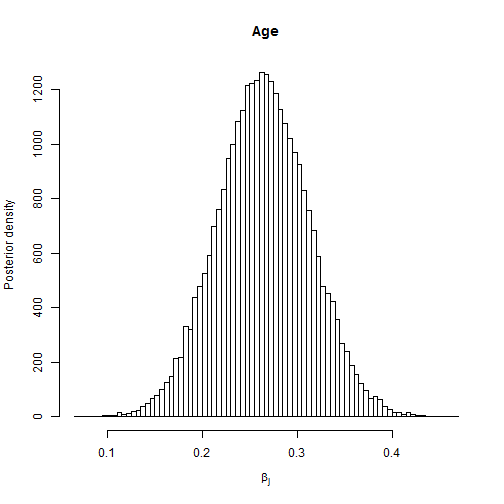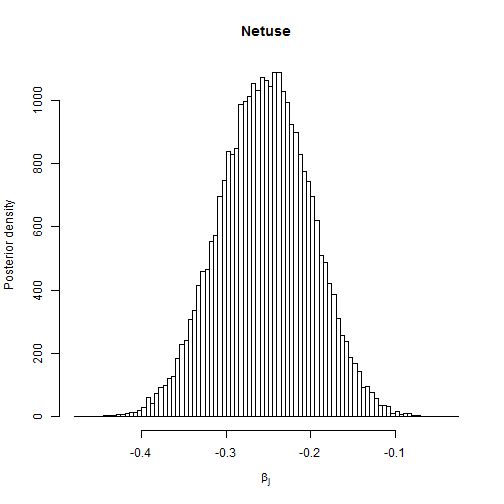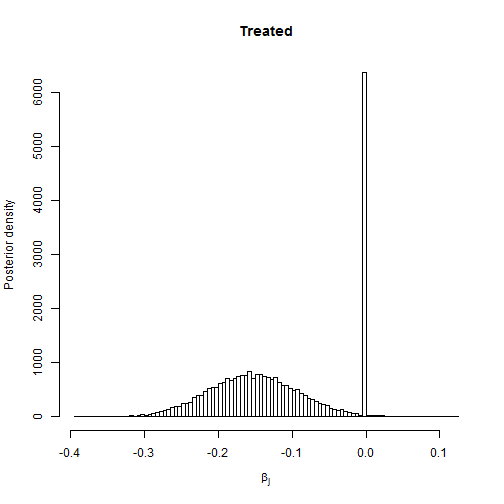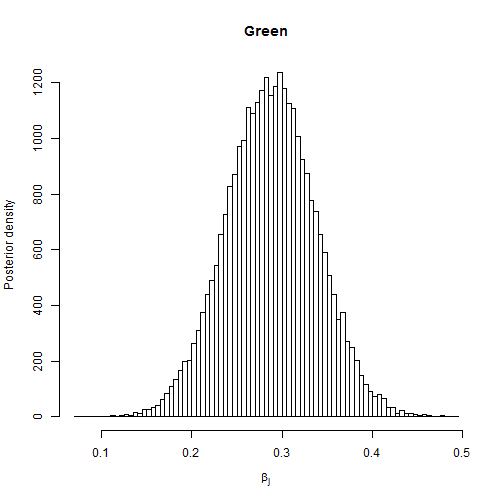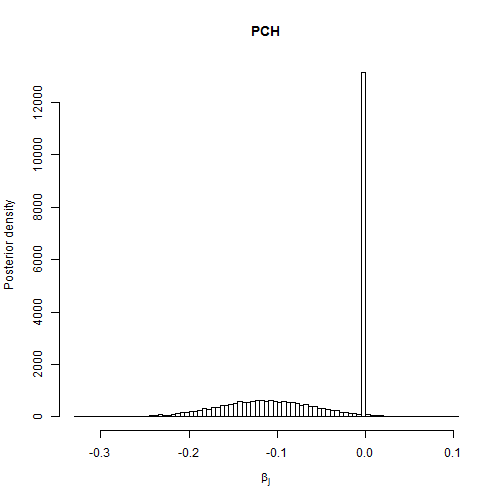Inc_Prob <- apply(beta!=0,2,mean)
Q        <- t(apply(beta,2,quantile,c(0.5,0.05,0.95)))
out      <- cbind(Inc_Prob,Q)

kable(round(out,2))

Inc_Prob 50% 5% 95%
Age 1.00 0.26 0.19 0.34
Netuse 1.00 -0.25 -0.34 -0.17
Treated 0.79 -0.13 -0.24 0.00
Green 1.00 0.29 0.21 0.37
PCH 0.56 -0.05 -0.19 0.00

Summary: Age, bed-net use and greenness are included with posterior probability one and are thus clearly important predictors of malaria. Treatment of the bed net and proximity to a health center are included with posterior probability more than a half and so there is moderate evidence that these variables are important predictors of malaria prevalence. The posterior distribution of these parameters has an unusual shape: they are a combination of a Gaussian curve for samples that include the variable and a spike at zero for samples that exclude the variable.

## Compute the posterior probability of each model

   model <- "Intercept"
for(j in 1:5){
model <- paste(model,ifelse(beta[,j]==0,"","+"))
model <- paste(model,ifelse(beta[,j]==0,"",names[j]))
}
model[1:5]

##  "Intercept + Age + Netuse + Treated + Green + PCH"
##  "Intercept + Age + Netuse + Treated + Green  "
##  "Intercept + Age + Netuse + Treated + Green + PCH"
##  "Intercept + Age + Netuse + Treated + Green  "
##  "Intercept + Age + Netuse + Treated + Green + PCH"

   beta[1:5,]

##            Age     Netuse    Treated     Green         PCH
## [1,] 0.2527252 -0.3024030 -0.1315871 0.2947381 -0.01603794
## [2,] 0.1660592 -0.2622006 -0.1285444 0.2221916  0.00000000
## [3,] 0.2250195 -0.1569258 -0.1485445 0.2938200 -0.12117986
## [4,] 0.2628377 -0.2609074 -0.1929870 0.3536249  0.00000000
## [5,] 0.2372177 -0.2419001 -0.1116356 0.2404865 -0.16442993

   model_probs <- table(model)/length(model)
model_probs <- sort(model_probs,dec=T)
round(model_probs,3)

## model
##     Intercept + Age + Netuse + Treated + Green
##                                            0.420
## Intercept + Age + Netuse + Treated + Green + PCH
##                                            0.370
##         Intercept + Age + Netuse   + Green + PCH
##                                            0.195
##             Intercept + Age + Netuse   + Green
##                                            0.015


Summary: Three models dominate the posterior probability:

1. Intercept + Age + Netuse + Green + Treated

2. Intercept + Age + Netuse + Green + Treated + PCH

3. Intercept + Age + Netuse + Green + PCH

Therefore it is clear that age, bed net use and greeness should be included in the model, but uncertainty about whether one or both of the remaining two variables should be included.